#### Thank you for registering.

One of our academic counsellors will contact you within 1 working day.

Please check your email for login details.

Click to Chat

1800-1023-196

+91-120-4616500

CART 0

• 0
MY CART (5)

Use Coupon: CART20 and get 20% off on all online Study Material

ITEM
DETAILS
MRP
DISCOUNT
FINAL PRICE
Total Price: Rs.

There are no items in this cart.
Continue Shopping• Complete JEE Main/Advanced Course and Test Series
• Offered Price: Rs. 15,900
• View Details

# Chapter 15: Areas Related To Circles Exercise – 15.4

### Question: 1

AB is a chord of a circle with centre O and radius 4 cm. AB is length 4 cm and divides circle into two segments. Find the area of minor segment

### Solution:Radius of circle r = 4cm = OA = OB

Length of chord AB = 4cm

OAB is equilateral triangle ∠AOB = 60° → θ

Angle subtended at centre θ = 60°

Area of segment (shaded region) = (Area of sector) - (Area of ∆ AOB)### Question: 2

A chord of circle of radius 14 cm makes a light angle at the centre. Find the areas of minor and major segments of the circle.

### Solution:

Radius (r) = 14 cmθ = 90°

= OA = OB

Area of minor segment (ANB) = (Area of ANB sector) - (Area of MOB)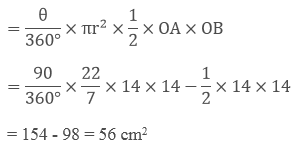Area of major segment (other than shaded) = Area of circle - Area of segment ANB

= πr2 - 56

= 22/7 × 14 × 14 - 56

= 616 - 56

= 560 cm2.

### Question: 3

A chord 10 cm long is drawn in a circle whose radius is 5√2 cm. Find the area of both segments

### Solution:

Given radius = r = 5√2 cm= OA = OB

Length of chord AB = 10 cm

In ∠OAB,

OA = OB = 5√2 cm AB = 10 cm

OA2 + OB2 = (5√2)2 + (5√2)2

= 50 + 50 = 100 = (AB)2

Pythagoras theorem is satisfied OAB is light triangle

θ = Angle subtended by chord = ∠AOB = 90°

Area of segment (minor) = Shaded region = Area of sector - Area of ∆OAB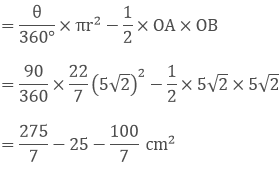Area of major segment = (Area of circle) - (Area of minor segment)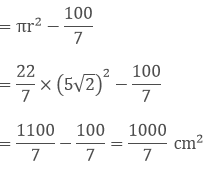### Question: 4

A chord AB of circle, of radius 14cm makes an angle of 60° at the centre. Find the area of minor segment of circle.

### Solution:

Given radius (r) = 14 cm = OA = OB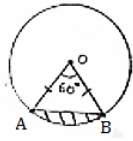θ = angle at centre = 60°

In AAOB, ∠A = ∠B [angles opposite to equal sides OA and OB] = x

By angle sum property ∠A + ∠B + ∠O = 180°

x + x + 60° = 180°

⟹ 2x = 120°

⟹ x = 60°

All angles are 60°, OAB is equilateral OA = OB = AB

Area of segment = Area of sector - Area. ∆le OAB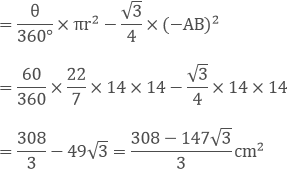### Question: 5

AB is the diameter of a circle, centre O. C is a point on the circumference such that ∠COB = θ. The area of the minor segment cutoff by AC is equal to twice the area of sector BOC. Prove that### Solution:

Given AB is diameter of circle with centre O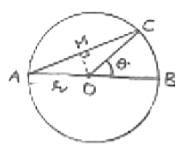∠COB = θ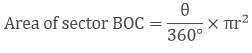Area of segment cut off, by AC = (Area of sector) - (Area of ∆AOC)

∠AOC = 180 - θ [∠AOC and ∠BOC form linear pair]Area of segment by AC = 2 (Area of sector BDC)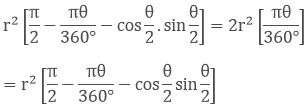Area of segment by AC = 2 (Area of sector BDC)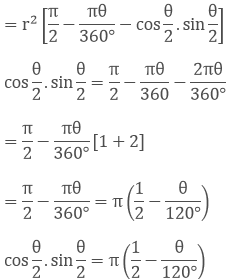### Question: 6

A chord of a circle subtends an angle O at the centre of circle. The area of the minor segment cut off by the chord is one eighth of the area of circle. Prove that 8 sin θ/2. cos θ/2 + π = π θ/45.

### Solution:

Let radius of circle = r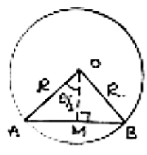Area of circle = πr2

AB is a chord, OA, OB are joined drop OM ⊥ AR This OM bisects AB as well as ∠AOB.

∠AOM = ∠MOB = 1/2(0) = θ/2 AB = 2AM

In ∆AOM, ∠AMO = 90°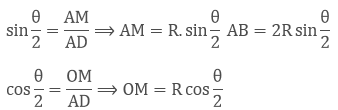Area of segment cut off by AB = (Area of sector) - (Area of triangles)Area of segment = 1/2 (Area of circle)## Complete JEE Main/Advanced Course and Test Series

OFFERED PRICE: Rs. 15,900
View Details Xpress Buy

### Course Features

• 731 Video Lectures
• Revision Notes
• Test paper with Video Solution
• Mind Map
• Study Planner
• NCERT Solutions
• Discussion Forum
• Previous Year Papers

### Course Features

• 728 Video Lectures
• Revision Notes
• Previous Year Papers
• Mind Map
• Study Planner
• NCERT Solutions
• Discussion Forum
• Test paper with Video Solution